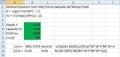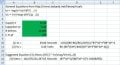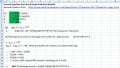# Implement RC Time Constant Math using a Spreadsheet

Thread Starter

#### Xerox

Joined Aug 6, 2015
13
I found the site http://www.ladyada.net/library/rccalc very useful as a machine calculator but I wanted to implement its equations on a spreadsheet which I did. The equations work fine for a capacitor start voltage of 0V finishing at Vc but what would the general equations and spreadsheet look like if the start voltage across the capacitor (Vc) equaled the supply voltage (V) and the capacitor was required to discharge to a required voltage.#### ericgibbs

Joined Jan 29, 2010
12,902
hi X,
I use this equation
tSecs= (R/C) * -ln(Vcap/Vinit)
Try it, see if it suits your app.
E

Thread Starter

#### Xerox

Joined Aug 6, 2015
13
I use this equation tSecs= (R/C) * -ln(Vcap/Vinit)
E
Hi Eric

Its bouncing it down here in Yorkshire just the sort of day to post a maths question. I tried your equation but it doesn't seem to work. Transposing transcendental math is a challenge at the best of times and coding it is something else. In my initial post some of the cell references were wrong and I've changed them.Thread Starter

#### Xerox

Joined Aug 6, 2015
13
hi X,
The equation works OK for me.
The clouds are back and its cold and overcast just to sort of weather to do some thinking.
When I put your equation into a spreadsheet I got 0.18 seconds which is good enough so I went for perfection figuring the charge and discharge times should be symmetrical. With the help of a physics tutorial I came up with this:•ericgibbs
Thread Starter

#### Xerox

Joined Aug 6, 2015
13
Similar threads